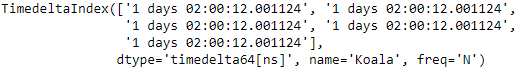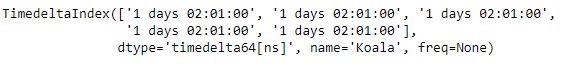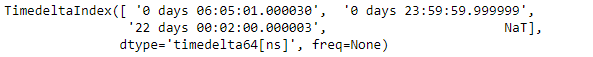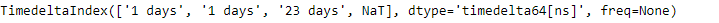Skip to content
Related Articles
Python | Pandas TimedeltaIndex.ceil
• Last Updated : 28 Dec, 2018

Python is a great language for doing data analysis, primarily because of the fantastic ecosystem of data-centric python packages. Pandas is one of those packages and makes importing and analyzing data much easier.

Pandas` TimedeltaIndex.ceil()` function ceil the index of the TimedeltaIndex object to the specified freq. The function takes the frequency as an argument to which we wan to ceil the values.

Syntax : TimedeltaIndex.ceil(freq)

Parameters :
freq : freq string/object

Return : index of same type

Example #1: Use `TimedeltaIndex.ceil()` function to ceil the value of the given TimedeltaIndex object to the specified frequency.

 `# importing pandas as pd``import` `pandas as pd`` ` `# Create the first TimedeltaIndex object``tidx ``=` `pd.TimedeltaIndex(start ``=``'1 days 02:00:12.001124'``, periods ``=` `5``,``                                            ``freq ``=``'N'``, name ``=``'Koala'``)`` ` `# Print the TimedeltaIndex object``print``(tidx)`

Output :Now we will use the `TimedeltaIndex.ceil()` function to ceil the value to minutely frequency.

 `# ceil the values to minutely frequency.``tidx.ceil(``'T'``)`

Output :As we can see in the output, the `TimedeltaIndex.ceil()` function has returned a new index object containing values ceiled to the desired frequency.

Example #2: Use `TimedeltaIndex.ceil()` function to ceil the value of the given TimedeltaIndex object to the specified frequency.

 `# importing pandas as pd``import` `pandas as pd`` ` `# Create the TimedeltaIndex object``tidx ``=` `pd.TimedeltaIndex(data ``=``[``'06:05:01.000030'``, ``'+23:59:59.999999'``,``                                      ``'22 day 2 min 3us 10ns'``, ``None``])`` ` `# Print the TimedeltaIndex object``print``(tidx)`

Output :Now we will use the `TimedeltaIndex.ceil()` function to ceil the value to daily frequency.

 `# ceil the values to daily frequency.``tidx.ceil(``'D'``)`

Output :As we can see in the output, the `TimedeltaIndex.ceil()` function has returned a new index object containing values ceiled to the desired frequency.

Attention geek! Strengthen your foundations with the Python Programming Foundation Course and learn the basics.

To begin with, your interview preparations Enhance your Data Structures concepts with the Python DS Course. And to begin with your Machine Learning Journey, join the Machine Learning – Basic Level Course

My Personal Notes arrow_drop_up